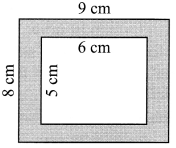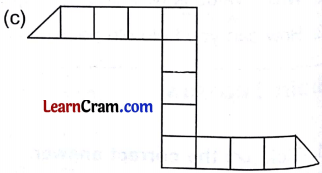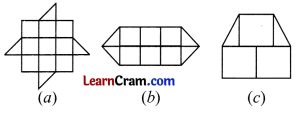# DAV Class 4 Maths Chapter 12 Brain Teasers Solutions

The DAV Maths Book Class 4 Solutions and DAV Class 4 Maths Chapter 12 Brain Teasers Solutions of Area offer comprehensive answers to textbook questions.

## DAV Class 4 Maths Ch 12 Brain Teasers Solutions

Question 1.
(a) The area of the shaded portion is(i) 72 sq. cm
(ii) 30 sq. cm
(iii) 102 sq.cm
(iv) 42 sq .cm
Area of shaded portion = Area of outer rectangle – Area of inner rectangle
Area of outer rectangle = 9 cm × 8 cm = 72 sq. cm
Area of inner rectangle = 5 cm × 6 cm = 30 sq. cm
Area of shaded portion = 72 cm2 – 30 cm2
= 42 sq. cm
Hence, option (iv) is correct.

(b) Length of a rectangle is 12 cm and its breadth is 4 cm less than the length. The area of the rectangle is –
(i) 40 sq. cm
(ii) 96 sq. cm
(iii) 40 cm
(iv) 96 cm
Length of rectangle = 12 cm
Breadth of rectangle = L – 4 cm
= 12 – 4
= 8 cm
so, Area of rectangle = 12 cm × 8 cm
= 96 sq. cm
Hence, option (ii) is correct.

(c) The best unit to measure area is:
(i) rectangle
(ii) square
(iii) triangle
(iv) circle
(ii) square

(d) Area of the given figure is squares.(i) 6
(ii) 10
(iii) 12
(iv) 5
(i) 6Hence option (i) is correct.

(e) Area of a rectangle of length 7 cm and breadth 4 cm is
(i) 28 cm
(ii) 22 sq. cm
(iii) 22 cm
(iv) 28 sq. cm
Area = 7 cm × 4cm
= 28 sq. cm
Hence option (iv) is correct.Question 2.
Which has more area?
(a) Postcard or Inland letter
(b) Blackboard or art sheet
(c) 10 rupee note or a 100 rupee note
(d) Handkerchief or Bedsheet.
(a) Inland letter
(b) Blackboard
(c) 100 rupee note
(d) Bedsheet

Question 3.
Find the area in terms of unit squares.11 squares.4 squares.12 Squares.

Question 4.
Ramu has a paddy field which is in the shape of a rectangle. It has a length of 15 m and breadth of 8 m. If he has ploughed an area of 90 sq. m of the field, how much more area is to be ploughed?
Length of paddy field = 15 m
= 15 m × 8 m
= 120 m2.
He has ploughed = 90 sq. m.
Area left for ploughing= 120 sq. m – 90 sq. m
= 30 sq. m
Thus, 30 sq. m area is to be ploughed.Question 5.
A square park is to be watered. If one side of the park is 5 m, find the area to be watered?
One side of square park = 5 m
Area of square park = side × side
= 5 m × 5 m
= 25 sq. m

Question 6.
A wooden sheet is 15 m long and 6 m broad. It has to be painted on both sides. Find the cost of painting at ₹ 30 per square metre.
Length of wooden sheet = 15 m
Breadth of wooden sheet = 6 m
Area = 15 m × 6 m
= 90 sq. m
∴ Area of both sides = 2 × 90 sq. m
= 180 sq.m
Painting cost of 1 sq. m = ₹ 30
∴ Painting cost of 180 sq. m = 30 × 180
= ₹ 5,400

Question 1.
Tick (✓) the figure which has smaller surface.Question 2.
Find the area of these figure in term of unit area.(a) 11 squares
(b) 8 squares
(c) 4 squares

Question 3.
Find the area of the rectangles whose dimensions are given below.
(a) Length = 24m Breadth =15m
Area of rectangle = Length × Breadth
= 24 m × 15 m
= 360 sq. m.

(b) Length = 15 m Breadth = 50cm
Area of rectangle = Length × Breadth
Area of rectangle = 15 m ×x 0.50 m
= 7.50 sq. m

(c) Length = 170 cm Breadth = 50 cm
Area of rectangle = Length × Breadth
Area of rectangle = 170 × 50
= 8500 sq. mQuestion 4.
Find the area of shaded portion.(a) Area of shaded portion of rectangle = Area of outer rectangle – Area of inner rectangle
Area of outer rectangle = Length × Breadth
= 15 cm × 10 cm
= 150 sq. cm

Area of inner rectangle = Length × Breadth
= 9 cm × 4 cm
= 36 sq. cm

Area of shaded portion =150 sq. cm – 36 sq. cm
= 114 sq. cm

(b) Area of shaded portion of square = Area of outer square – Area of inner square
= (10 × 10) -(7 × 7)
= 100 sq. cm – 49 sq. cm
= 51 sq. cm

Question 5.
The length of a playground is 15 metres and breadth is 9 metres. Find the area of the playground.